EXERCISE 7.4:

1). Write each fraction. Arrange them in ascending and descending order using correct sign (<), (=), (>). between the fraction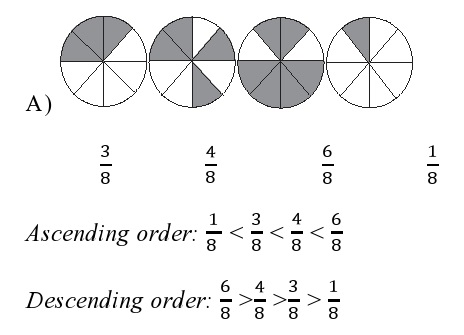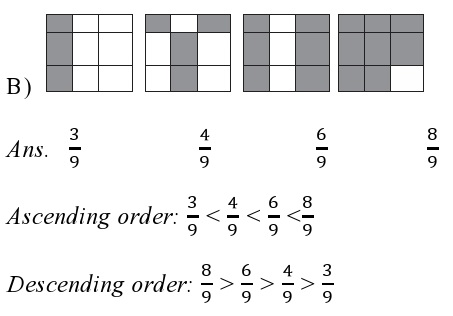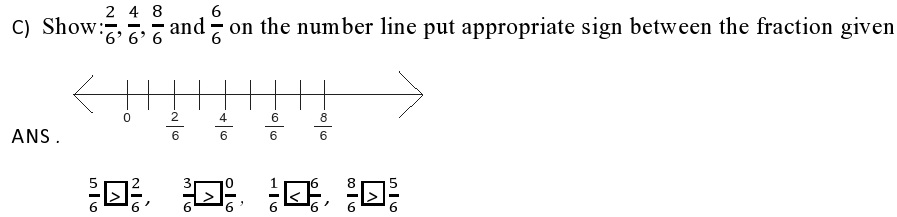2). Compare the fraction and put an appropriate sign.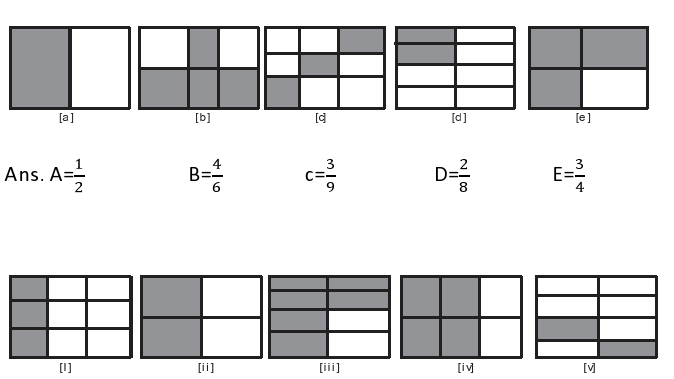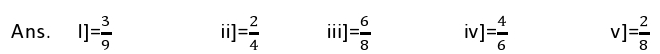Pairing up
[a]=[ii], [b]=[iv], [c]=[i], [d]=[v], [e]=[vi]

3). Make five more such pairs and put appropriate signs.
Ans:
i) 4/9  <  7/9
ii)1/7  <  1/5
iii) 7/13  <  11/13
iv) 2/4  =  3/6
v) 1/5  <  1/2

4). Name the fraction and arrange them in ascending order: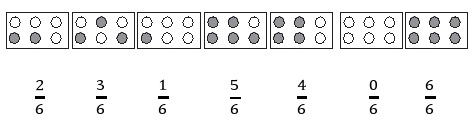Ans: Ascending order: 0/6 < 1/6 <2/6 <3/6 <4/6 <5/6 <6/6

5). Look at the figure and write (<) or (>) , (=) between the pairs of fraction
Ans:
a) 1/6  (<)  1/3
b)3/4  (>)  2/6
c)2/3  (>)  2/4
d)6/6  (=)  3/3
e)0/1  (=)  0/6
f) 5/6  (<)  5/5
Make five more such problems and solve them with your friends
a)1/2  (>)  3/4
b) 2/2  (=)  5/5
c) 2/4  (<)  1/3
d) 4/5  (<)  3/4
e) 4/6  (<)  1/3

6). How quickly can you do this? Fill appropriate signs ( < , = , > )
Ans:
a) 1/2  {>}  1/5              b) 2/4 {=} 3/6        c) 3/5 {<} 2/3        d) 3/4 {>} 3/8
e) 3/5  {<}  6/5             f) 7/9 {>}  3/9        g) 1/4 {=} 2/8         h) 6/10 {<} 4/5
i) 3/4  {<} 7/8              j) 6/10 {<}  4/5      k) 5/7 {=} 15/21

7). The following fractions represent just three different numbers separate them into three groups of equivalent fraction, by changing each one to its simplest form.
Ans:
a) 2/12 = 1/6                                     b) 3/15 = 1/5                                           c) 8/50 = 4/25
d) 16/100 = 4/25                             e) 10/60 = 1/6                                         f) 15/75 = 1/5
g) 12/(60 ) = 1/5                             h) 16/96 = 1/6                                          i) 12/75 = 4/25
j) 12/72 = ( 1)/6                              k) 3/18 = 1/6                                             l) 4/25 = 4/25

8). Find answers to the following. Write and indicate how you solved them
a) Is 5/9 equal to 4/5 ?
Ans:
(5×5)/(9×5) = 25/45  and  (4×9)/(5×9) = 36/45                                     [LCM of 9 and 5 is 45]
25/45 ≠ 36/45
5/9 ≠ 4/5
b) Is 9/16 equal to 5/9
Ans. (9×9)/(16×9) = 81/144  and  (5×16)/(9×16) = 80/( 144)              [LCM of 16 and 9 is 144]
81/144≠ 80/144
9/16 ≠5/9
c) Is 4/5 equal to 16/20
Ans. (4×20)/(5×20) = 80/100 and (16×5)/(20×5) = 80/100             [LCM of 5 and 20 is 100]
80/100 = 80/100
4/5 = 16/20
d) Is 1/15 equal to 4/30 ?
Ans. (1×2)/(15×2) = 2/30  and  (4×1)/(30×1) = 4/30                            [LCM of 15 and 30 is 30]
2/30 ≠4/30 1/15 ≠ 4/30

9). Ila reads 25 pages of a book containing 100 pages lalita reads 1/2 of the same book who reads less
Ans. Ila reads 25 pages out of 100 pages
i.e. fraction of reading = 25/100 = 1/4
Since 1/4 < 1/2